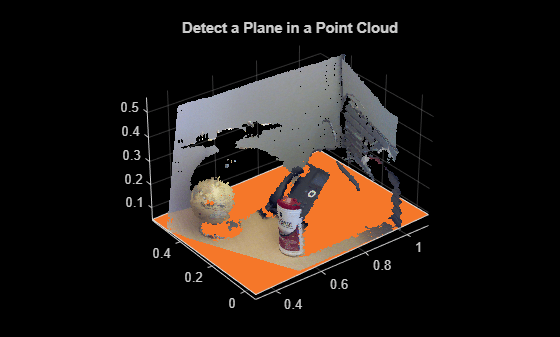# plot

Plot plane in a figure window

## Syntax

``plot(model)``
``plot(model,Name,Value)``

## Description

example

````H` = `plot(model)` plots a plane within the axis limits of the current figure. `H` is the handle to the `patch`.```
````H` = `plot(model,Name,Value)` includes additional options specified by one or more `Name,Value` pair arguments.```

## Examples

collapse all

`load('object3d.mat');`

Display the point cloud.

```figure pcshow(ptCloud) xlabel('X(m)') ylabel('Y(m)') zlabel('Z(m)') title('Detect a plane in a point cloud')```Set the maximum point-to-plane distance (2cm) for plane fitting.

`maxDistance = 0.02;`

Set the normal vector of a plane.

`referenceVector = [0, 0, 1];`

Set the maximum angular distance (5 degrees).

`maxAngularDistance = 5;`

Detect the table in the point cloud and extract it.

`model = pcfitplane(ptCloud,maxDistance,referenceVector,maxAngularDistance);`

Plot the plane.

```hold on plot(model)```## Input Arguments

collapse all

Parametric plane model returned by `planeModel`.

### Name-Value Arguments

Specify optional comma-separated pairs of `Name,Value` arguments. `Name` is the argument name and `Value` is the corresponding value. `Name` must appear inside quotes. You can specify several name and value pair arguments in any order as `Name1,Value1,...,NameN,ValueN`.

Example: `'Color'`, `'r'`.

Output axes, specified as the current axes for displaying the visualization.

Color of the plane, specified as a 1-by-3 RGB vector or a short or long color name.

Color NameShort NameRGB TripletAppearance
`'red'``'r'``[1 0 0]``'green'``'g'``[0 1 0]``'blue'``'b'``[0 0 1]``'cyan'` `'c'``[0 1 1]``'magenta'``'m'``[1 0 1]``'yellow'``'y'``[1 1 0]``'black'``'k'``[0 0 0]``'white'``'w'``[1 1 1]`Introduced in R2015b